Math Concepts
How to Teach Addition to Kids
13.5k views

 1 What is Addition? 2 How to Teach Addition to kids? 3 How to teach Addition with Activities? 4 Addition for kids with Activity Log 5 Summary 6 Frequently Asked Questions (FAQs) 7 External References

5 January 2021

Addition can be visualized as 'putting together' or two or more quantities. The addition is taking two or more numbers and adding them together. The sign for Addition is + (plus). This is one of the four arithmetic operations.

[Or]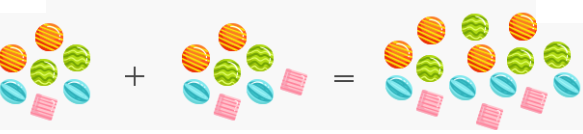## How to Teach Addition to Kids?

It is important for children to see how when we put together two quantities it gives a larger group.

•  Ask children to perform addition using real-life objects like pens, pencils, chocolates, marbles.

• Use math manipulatives like colour counters, abacus, flats, rods and blocks to help children perform addition.

• Pop quiz: you can ask children 5 rapid-fire questions on addition.

• Based on the pocket money children receive Ask children to maintain a log of their expenses. At the end of the month, you can ask for the remaining amount.

• Use flats, rods and blocks to show how the procedure works with larger numbers.

Count how many orange balls in each rowAdding two numbers with orange balls### Addition for kids using the number strips

Example 1

1. You can use a number strip to count forward.

2. Add 2 and 4 by counting forward.

3. Start at 2 and count 4 boxes forward and you reach at 6.0 1 2 3 4 5 6 7 8 9
 2+4=6

Example 2

1. You can use a number strip to count forward.

2. Add 3 and 3 by counting forward.

3. Start at 3 and count 3 boxes forward and you reach at 6.0 1 2 3 4 5 6 7 8 9
 3+3=6

### Addition for Kids with Zero

The addition property of 0 states that for any number being added to zero, the sum is the same number.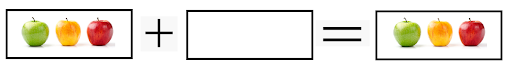3 Apples                                        Zero Apples                                           3 Apples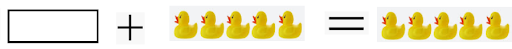Zero Ducks                                       5 Ducks                                                        5 Ducks

 When zero is added to any number or when any number is added to zero, is equal to the number itself.

###4                                +           1              =                                   51               +                              4                                    =                          5

 When you add one (1) to a number or when 1 is added to a number, we get the next number as the answer.

### Addition for kids with two numbers

Example 1

 1st Number 5 2nd Number 3 Total (+) 8

Steps to be followed

Step 1: Take the first number 5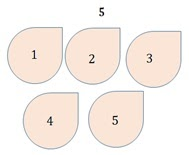Step 2: As the second number is 3, take the first number 5 and then count 3 more that is 6, 7, and 8.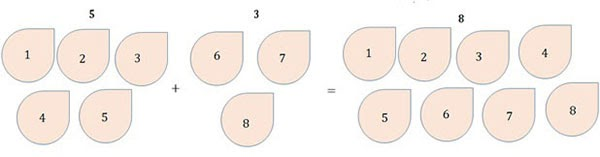We get the answer as 8.

Example 2:

 1st Number 7 2nd Number 4 Total (+) 11

Steps to be followed

Step 1: Take the first number 7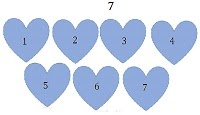Step 2: As the second number is 4, take the first number 7 and then count 4 more that is, 8, 9, 10, and 11.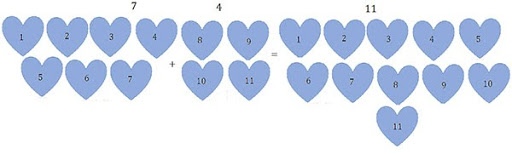## How to Teach Addition with Activities?

When kids are frustrated with the concept of addition.

Addition can be taught with activities, which helps kids to grasp the concept more quickly with a free mindset.

Activities such as pictorial problems, mobile games and outdoor activities can be used to teach addition.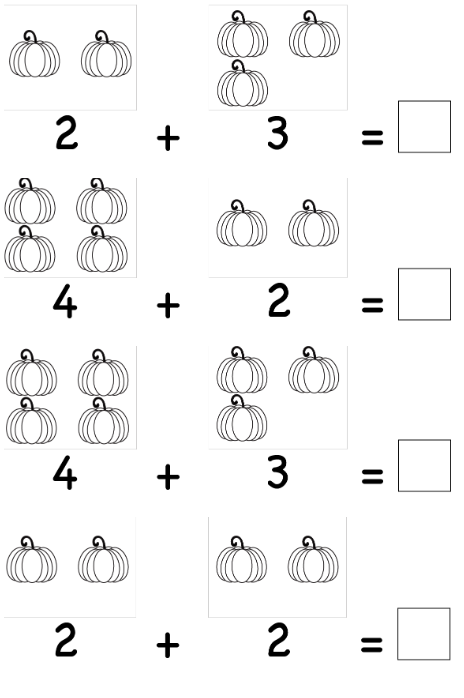Q: Ramu has 6 apples and Somu has 9 apples. How many apples in all?

A:

Ramu has 6 applesSomu has 9 apples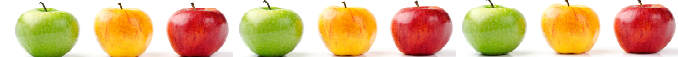Total number of Apples are 6 + 9 = 15

Q: In a class, there are 8 boys and 7 girls. How many students are there in all?

A:

Number of boys in class 4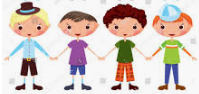Number of girls in class 8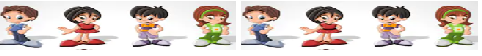Total number of students in class 4+ 8 = 12

## Addition for Kids with Activity log

### Ordering in Addition with a single-digit

When you change the order of 2 or more numbers while adding, the answer remains the same.

2 + 4 = 6  and 4 + 2 = 6

1 + 2 = 3  and 2 + 1 = 3

2 + 5 = 7  and 5 + 2 = 7

### Addition for kids with two-digit numbers without carrying

When we add two-digit numbers, we need to add one’s place digits first and then ten’s place digits.

Example 1

25 + 31 = ?

 Tens Place One’s place 1st Number 2 5 2nd Number 3 1 Total (+) 5 6

Steps to be followed

Step 1: Add the digits in one’s place (5 + 1 = 6)

Step 2: Add the digits in ten’s place  (2 + 3 = 5)

Step 3: Place the total of one’s place digits in one’s place and the total of ten’s place digits in ten’s place. Now we have 5 Tens and 6 Ones which makes the sum as 56.

Example 2

29 + 90 = ?

 Tens Place One’s place 1st Number 2 9 2nd Number 9 0 Total (+) 11 9

Step 1: Add the digits in one’s place (9 + 0 = 9)Steps to be followed

Step 2: Add the digits in ten’s place  (2 + 9 = 11)

Step 3: Place the total of one’s place digits in one’s place and the total of ten’s place digits in ten’s place. Now we have 11 tens and 9 ones, which sums to 119.

### Addition for kids with two-digit numbers without carrying

When we add two-digit numbers, we need to add one’s place digits first and then ten’s place digits. If the numbers in the one’s place are added we get the sum as a two-digit number which is more than 9 then that number contains tens and ones. Below are a few examples of how to split if the added number is more than 9.

• 9 + 2 = 11 we need to split as 1 ten + 1 one

• 8 + 6 = 14 we need to split as 1 ten + 4 ones

• 6 + 4 = 10 we need to split as 1 ten + 0 ones

Example 1

 Ten’s place One’s place carryover 1 First Number 3 9 2nd Number 4 3 Total (+) 8 2

Step 1: Add the digits in one’s place (9 + 3 = 12, we need to split as 1 ten + 2 ones). So we have placed 2 in one’s place and 1 in ten’s place as a carryover (in the above addition, carryover is marked in red color.Steps to be followed

Step 2: Add the digits in ten’s place  (3 + 4 + 1 (carry) = 8

Step 3: Place a total of one’s place digits in one’s place and a total of ten’s place digits in ten’s place that means 82 is the answer.

Example 2

 Ten’s place One’s place carryover 1 First Number 7 7 2nd Number 6 6 Total (+) 14 3

Step 1: Add the digits in one’s place (7 + 6 = 13, we need to split as 1 ten + 3 ones). So we have placed 3 in one’s place and 1 in ten’s place as a carryover (in the above addition, carryover is marked in red color.Steps to be followed

Step 2: Add the digits in ten’s place  (7 + 6 + 1 (carryover) = 14)

Step 3: Place the total of one’s place digits in one’s place and the total of ten’s place digits in ten’s place. Now we have 14 tens and 3 ones, which sums to 143.

### Ordering in Addition with two-digit

When you change the order of 2 or more numbers while adding, the answer remains the same.

20 + 40 = 60  and 40 + 20 = 60

11 + 22 = 33  and 22 + 11 = 33

13 + 17 = 30  and 17 + 13 = 30

The same concept could be applied while adding any number of digits.

## Summary

• The addition is taking two or more numbers and adding them together

• Adding any number to zero results in the number itself

• Adding any number with one results in the next number

• Adding numbers in any order the result is the same.

Cuemath, a student-friendly mathematics and coding platform, conducts regular Online Live Classes for academics and skill-development, and their Mental Math App, on both iOS and Android, is a one-stop solution for kids to develop multiple skills. Understand the Cuemath Fee structure and sign up for a free trial.

## How to teach addition to kids?

It is important for children to see how when we put together two quantities it gives a larger group.

•  Ask children to perform addition using real-life objects like pens, pencils, chocolates, marbles.

• Use math manipulatives like colour counters, abacus, flats, rods and blocks to help children perform addition.

• Pop quiz: you can ask children 5 rapid-fire questions on addition.

• Based on the pocket money children receive Ask children to maintain a log of their expenses. At the end of the month, you can ask for the remaining amount.

• Use flats, rods and blocks to show how the procedure works with larger numbers.

Related Articles